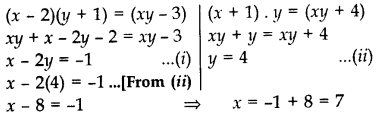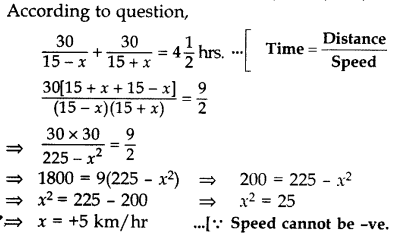# CBSE Solutions for Class 10 Maths

#### Select CBSE Solutions for class 10 Subject & Chapters Wise :

Identify whether they have One Solution, Infinite Solutions or No Solutions :-

3y+4x=6 , 12y+16x=30

Answer :

Infinite Solutions

Identify whether they have One Solution, Infinite Solutions or No Solutions :- y+2x=10 , 3y+6x=30

Answer :

One Solution

Identify whether they have One Solution, Infinite Solutions or No Solutions :- 7y−11x=53 ,19y−17x=456

Answer :

No Solutions

Identify whether they have One Solution, Infinite Solutions or No Solutions :- y=7,x=−2

Answer :

One Solution

Identify whether they have One Solution, Infinite Solutions or No Solutions :- ay+bx=a−b , by−ax=a+b

Answer :

One Solution

Identify whether they have One Solution, Infinite Solutions or No Solutions :- 2y+3x=0, 124y+13x=0

Answer :

One Solution

Identify whether they have One Solution, Infinite Solutions or No Solutions :- x=11 ,x=−11

Answer :

One Solution

Identify whether they have One Solution, Infinite Solutions or No Solutions :-  152y−378x=−74 , −378y+152x=−604

Answer :

No Solutions

Using substitution method solve the equation :- x−2y+300=0, 6x−y−70=0

Answer :

x=2y−300 ----(1)
6x−y−70=0----(2)
Substituting value from (1) to (2)
6(2y−300)−y−70=06(2y−300)−y−70=0
=> y=170y=170
Putting this in (1)
x=40

Using substitution method solve the equation :- 5x−y=5, 3x−y=3

Answer :

5x−y=5 ----(1)
3x−y=3 ----(2)
Substituting value from (1) to (2)
3x + 5 -5x = 3
->   x=1
Putting this in (1)
->    y=0

Using elimination method solve the equation :- x+y−40= ,7x+3y=180

Answer :

x+y−40=0  ---(A)
7x+3y=180  ---(B)
Multiplying equation (A) by 7
7x+7y−280=0 ---(C)
Subtracting equation (B) from equation (C)
We get
4y=100 => y=25
Substituting this in (A) ,we get x=15

Identify whether statement True or False :- 51x+68y=110 & 3x+4y=99 are consistent pair

Answer :

False

Identify whether statement True or False :- x−3y=3 & 3x−9y=2 are consistent pair

Answer :

False

Identify whether statement True or False :- x+6y=12 and 4x+24y=64 are inconsistent pair of equation

Answer :

True

Identify whether statement True or False :-  2x+6y=12 and 8x+24y=65 are consistent pair of equation

Answer :

False

Identify whether statement True or False :-  Lines represented by x−y=0 and x+y=0 are perpendicular to each other

Answer :

True

Identify whether statement True or False :-   x=0 ,y=0 has one unique solution

Answer :

True

Identify whether statement True or False :-  There are infinite solution for equation 17x+12y=30

Answer :

True

Identify whether statement True or False :-  Pair of lines 117x+14y=30 , 65x+11y=19 are consistent and have a unique solution

Answer :

True

Identify whether statement True or False :-  Line 4x+5y=0 and 11x+17y=0 both passes through origin

Answer :

True

A two digit number is seven times the sum of its digits. The number formed by reversing the digits is 18 less than the given number. Find the given number. (2013)

Answer :

Let unit’s place digit be x and ten’s place digit be y.
Then original number = x + 10y
and reversed number = 10x + y
According to the Question,
x + 10y = 7(x + y)
x + 10y = 7x + 7y
⇒ 10y – 7y = 7x – x
⇒ 3y = 6x ⇒ y = 2x …(A)
(x + 10y) – (10x + y) = 18
x + 10y – 10x – y = 18
⇒ 9y – 9x = 180
⇒ y – x = 2 …[Dividing by 9
⇒ 2x – x = 2 …[From (A)
∴ x = 2
Putting the value of ‘x’ in (A), we get y = 2(2) = 4
∴ Required number = x + 10y
= 2 + 10(4) = 42

The age of the father is twice the sum of the ages of his 2 children. After 20 years, his age will be equal to the sum of the ages of his children. Find the age of the father. (2012)

Answer :

Let the present ages of his children be x years and y years.
Then the present age of the father = 2(x + y) …(A)
After 20 years, his children’s ages will be
(x + 20) and (y + 20) years
After 20 years, father’s age will be 2(x + y) + 20
According to the Question,
2(x + y) + 20 = x + 20 + y + 20
2x + 2y + 20 = x + y + 40
2x + 2y – x – y = 40 – 20
x + y = 20 …[From (A)
Present age of father = 2(20) = 40 years

Sita Devi wants to make a rectangular pond on the road side for the purpose of providing drinking water for street animals. The area of the pond will be decreased by 3 square feet if its length is decreased by 2 ft. and breadth is increased by 1 ft. Its area will be increased by 4 square feet if the length is increased by 1 ft. and breadth remains same. Find the dimensions of the pond. (2014)

Answer :

Let, length of rectangular pond = x
breadth of rectangular pond = y
Area of rectangular pond = xy
According to Question,=>  Length of rectangular pond = 7 ft.
Breadth of rectangular pond = 4 ft.

On reversing the digits of a two digit number, number obtained is 9 less than three times the original number. If difference of these two numbers is 45, find the original number. (2014)

Answer :

Let unit’s place digit be x and ten’s place digit bey.
Original number = x + 10y Reversed number = 10x + y
According to the Question,
10x + y = 3(x + 10y) – 9

10x + y = 3x + 30y – 9
10x + y – 3x – 30y = -9
7x – 29y = -9 …(A)
10x + y – (x + 10y) = 45

9x – 9y = 45
x – y = 5 …[Dividing both sides by 9
x – 5 + y …(B)
Solving (
A),
7x – 29y = -9
7(5 + y) – 29y = -9 …[From (B)
35+ 7y – 29y = -9
-22y = -9 – 35
-22y = -44
y = 44/22 = 2
Putting the value of y in (B),
x = 5 + 2 = 7

Original number = x + 10y
= 7 + 10(2) = 27

Speed of a boat in still water is 15 km/h. It goes 30 km upstream and returns back at the same point in 4 hours 30 minutes. Find the speed of the stream. 2017D

Answer :

Let, speed of stream = x km/hr
Speed of boat in still water = 15 km/hr
then, the speed of the boat upstream = (15 – x) km/hr
and the speed of the boat downstream = (15 + x) km/hr=> Speed of stream = 5 km/hr

Coordinates of points on line x+y=0

 Co-ordintes of x Co-ordinates of y 1 1 A -7 2 3 B -1 3 5 C -3 4 7 D -5

Answer :

1-B, 2-C, 3-D, 4-A

Coordinates of points on line x-y=0

 Co-ordintes of x Co-ordinates of y 1 1 A 7 2 3 B 5 3 5 C 3 4 7 D 1

Answer :

1-D, 2-C, 3-B, 4-A

Coordinates of points on line 2x+y=0

 Co-ordintes of x Co-ordinates of y 1 4 A -4 2 -6 B -2 3 8 C 5 4 -10 D 3

Answer :

1-B,2-D,3-A, 4-C

Coordinates of points on line x+2y=0

 Co-ordintes of x Co-ordinates of y 1 -8 A 1 2 -2 B -2 3 6 C -3 4 4 D 4

Answer :

1-D, 2-A, 3-C, 4-B

Coordinates of points on line 2x-y=0

 Co-ordintes of x Co-ordinates of y 1 2 A 6 2 3 B 10 3 4 C 4 4 5 D 8

Answer :

1-C, 2-A, 3-D, 4-B

### Take a Test

Choose your Test :

### Chapter 3 : Pair of Linear Equations in Two Variables

Two linear equations in the same two variables are called pair of linear equations in two variables. The pair of linear equations in two variables can be represented graphically and algebraically. The graph can be represented by two lines:
• If the lines intersect at a point, the pair of equations is said to be consistent.
• If the lines coincide, the pair of equations is dependent.
• If the lines are parallel, the pair of equations is inconsistent.

### Browse & Download CBSE Books For Class 10 - All Subjects

The GSEB Books for class 10 are designed as per the syllabus followed Gujarat Secondary and Higher Secondary Education Board provides key detailed, and a through solutions to all the questions relating to the GSEB textbooks.

The purpose is to provide help to the students with their homework, preparing for the examinations and personal learning. These books are very helpful for the preparation of examination.

For more details about the GSEB books for Class 10, you can access the PDF which is as in the above given links for the same.